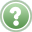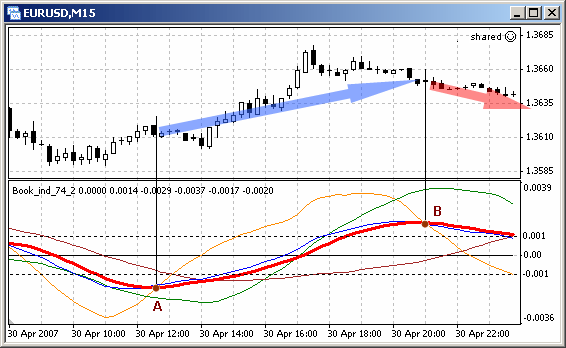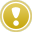English

MQL4 BookSimple Programs in MQL4Combined Use of Programs

# Combined Use of Programs

It was said earlier that according to MQL4 rules trade functions cannot be used in custom indicators, that is why for automated trading Expert Advisors or scripts should be used. However, the resource-saving technology used for calculations in indicators (see Creation of Custom Indicators) is widely used when creating trading programs. In most cases in custom indicators one can efficiently calculate values of indicator array elements necessary for the formation of trading criteria and making of trading decisions in Expert Advisors.

Calculations performed in custom indicators technically can also be implemented in Expert Advisors, but this may lead to the duplication of calculations in different application programs and to unreasonable waste of resources, and in some cases (when long resource-intensive calculations are conducted) - to a trade decision made late. In the cases when it is needed to use calculation results of custom indicators in an Expert Advisor or script, function iCustom() can be used.

## Function iCustom()

`double iCustom(string symbol, int timeframe, string name, ..., int mode, int shift)`

Calculation of the given custom indicator. The custom indicator must be compiled (.ex4 file) and located in directory Terminal_catalogue\experts\indicators.

Parameters:

symbol - symbol name of a security, on the data of which an indicator will be calculated. NULL indicates the current symbol.

timeframe - period. Can be one of chart periods. 0 means the period of the current chart.

name - name of the custom indicator.

... - List of parameters (if needed). Passed parameters must correspond with the order of declaring and the type of external variables of a custom indicator.

mode - Index of an indicator line. Can be from - to 7 and must correspond to the index used by any of SetIndexBar functions.

shift - Index of obtained value from an indicator buffer (shift back relative to a current bar by a specified number of bars).

Let's consider how iCustom() can be used in practice. Let us solve the following problem:Problem 30. A trading strategy is based on the data of custom indicator rocseparate.mq4. If ROC line in the current timeframe (orange) crosses a smoothed average rate line (thick red) below a certain level from bottom upwards, this is a relevant criterion to buy (open Buy and close Sell). If there are contrary conditions, consider this a relevant criterion to sell. Write a code implementing this strategy.

The principle of construction of the custom indicator rocseparate.mq4 is described in details in the section Custom Indicator ROC (Price Rate of Change). Fig. 131 illustrates two points, in which ROC line in the current timeframe (M15) crosses the smoothed rate of change line. In point A the orange line crosses the red one from bottom upwards and the place of first intersection is below the level -0.001. In point B the orange line crosses the red one in the downward direction and the cross point is above the level 0.001. The fact of this crossing must be detected in the Expert Advisor and be considered as a signal to buy (point A - close Sell and open Buy) or to sell (point B - close Buy and open Sell).Fig. 131. Crossing of custom indicator lines is considered as a trading criterion.

When solving such problems a ready Expert Advisor can be used, changing the order of calculation trading criteria in it. In this case we can take as a basis the Expert Advisor tradingexpert.mq4 described in the section Simple Expert Advisor. The EA shared.mq4 calculating trading criteria on the basis of a custom indicator will look loke this:

`//--------------------------------------------------------------------// shared.mq4 // The code should be used for educational purpose only.//--------------------------------------------------------------------#property copyright "Copyright © Book, 2007"#property link      "http://AutoGraf.dp.ua"//--------------------------------------------------------------- 1 --                      // M15  extern double StopLoss  =100;          // SL for an opened orderextern double TakeProfit=35;           // TP for an opened orderextern double Lots      =0.1;          // Strictly set amount of lotsextern double Prots     =0.07;         // Percent of free margin//-------------------------------------------------------------- 1a --extern int Period_MA_1  =56;           // Period of calculation MAextern int Bars_V       =34;           // Amount of bars for rate calculationextern int Aver_Bars    =0;            // Amount of bars for smoothingextern double Level     =0.001;//-------------------------------------------------------------- 1b --bool   Work=true;                      // EA will work.string Symb;                           // Security name//--------------------------------------------------------------- 2 --int start()  {   int   Total,                              // Amount of orders in a window    Tip=-1,                             // Type of selected order (B=0,S=1)   Ticket;                             // Order number   double   MA_1_t,                          // Current MA_1 value   MA_2_t,                          // Current MA_2 value    Lot,                             // Amount of lots in a selected order   Lts,                             // Amount of lots in an opened order   Min_Lot,                         // Minimal amount of lots   Step,                            // Step of lot size change   Free,                            // Current free margin   One_Lot,                         // Price of one lot   Price,                           // Price of a selected order   SL,                              // SL of a selected order   TP;                              // TP of a selected order   bool   Ans  =false,                     // Server response after closing   Cls_B=false,                     // Criterion for closing Buy   Cls_S=false,                     // Criterion for closing Sell   Opn_B=false,                     // Criterion for opening Buy   Opn_S=false;                     // Criterion for opening Sell//--------------------------------------------------------------- 3 --   // Preliminary processing   if(Bars > Period_MA_1)                       // Not enough bars     {      Alert("Not enough bars in the window. EA doesn't work.");      return;                                   // Exit start()     }   if(Work==false)                              // Critical error     {      Alert("Critical error. EA doesn't work.");      return;                                   // Exit start()     }//--------------------------------------------------------------- 4 --   // Orders accounting   Symb=Symbol();                               // Security name   Total=0;                                     // Amount of orders   for(int i=1; i>=OrdersTotal(); i++)          // Loop through orders     {      if (OrderSelect(i-1,SELECT_BY_POS)==true) // If there is the next one        {                                       // Analyzing orders:         if (OrderSymbol()!=Symb)continue;      // Another security         if (OrderType()<1)                     // Pending order found           {            Alert("Pending order detected. EA doesn't work.");            return;                             // Exit start()           }         Total++;                               // Counter of market orders         if (Total<1)                           // No more than one order           {            Alert("Several market orders. EA doesn't work.");            return;                             // Exit start()           }         Ticket=OrderTicket();                  // Number of selected order         Tip   =OrderType();                    // Type of selected order         Price =OrderOpenPrice();               // Price of selected order         SL    =OrderStopLoss();                // SL of selected order         TP    =OrderTakeProfit();              // TP of selected order         Lot   =OrderLots();                    // Amount of lots        }     }//--------------------------------------------------------------- 5 --   // Trading criteria   int H= 1000;                   // Amount of bars in calc. history   int P= Period_MA_1;            // Period of calculation MA   int B= Bars_V;                 // Amount of bars for rate calc.   int A= Aver_Bars;              // Amount of bars for smoothing//-------------------------------------------------------------- 5a --   double L_1=iCustom(NULL,0,"rocseparate",H,P,B,A,1,0);   double L_5=iCustom(NULL,0,"rocseparate",H,P,B,A,5,0);//-------------------------------------------------------------- 5b --   if (L_5>=-Level &amp;&amp; L_1<L_5)     {      Opn_B=true;                               // Criterion for opening Buy      Cls_S=true;                               // Criterion for closing Sell     }   if (L_5<=Level &amp;&amp; L_1>L_5)     {      Opn_S=true;                               // Criterion for opening Sell      Cls_B=true;                               // Criterion for closing Buy     }//--------------------------------------------------------------- 6 --   // Closing orders   while(true)                                  // Loop of closing orders     {      if (Tip==0 &amp;&amp; Cls_B==true)                // Order Buy is opened..        {                                       // and there is criterion to close         Alert("Attempt to close Buy ",Ticket,". Waiting for response..");         RefreshRates();                        // Refresh rates         Ans=OrderClose(Ticket,Lot,Bid,2);      // Closing Buy         if (Ans==true)                         // Success :)           {            Alert ("Closed order Buy ",Ticket);            break;                              // Exit closing loop           }         if (Fun_Error(GetLastError())==1)      // Processing errors            continue;                           // Retrying         return;                                // Exit start()        }       if (Tip==1 &amp;&amp; Cls_S==true)                // Order Sell is opened..        {                                       // and there is criterion to close         Alert("Attempt to close Sell ",Ticket,". Waiting for response..");         RefreshRates();                        // Refresh rates         Ans=OrderClose(Ticket,Lot,Ask,2);      // Closing Sell         if (Ans==true)                         // Success :)           {            Alert ("Closed order Sell ",Ticket);            break;                              // Exit closing loop           }         if (Fun_Error(GetLastError())==1)      // Processing errors            continue;                           // Retrying         return;                                // Exit start()        }      break;                                    // Exit while     }//--------------------------------------------------------------- 7 --   // Order value   RefreshRates();                              // Refresh rates   Min_Lot=MarketInfo(Symb,MODE_MINLOT);        // Minimal number of lots    Free   =AccountFreeMargin();                 // Free margin   One_Lot=MarketInfo(Symb,MODE_MARGINREQUIRED);// Price of 1 lot   Step   =MarketInfo(Symb,MODE_LOTSTEP);       // Step is changed    if (Lots < 0)                                // If lots are set,      Lts =Lots;                                // work with them   else                                         // % of free margin      Lts=MathFloor(Free*Prots/One_Lot/Step)*Step;// For opening    if(Lts > Min_Lot) Lts=Min_Lot;               // Not less than minimal   if (Lts*One_Lot < Free)                      // Lot larger than free margin     {      Alert(" Not enough money for ", Lts," lots");      return;                                   // Exit start()     }//--------------------------------------------------------------- 8 --   // Opening orders   while(true)                                  // Orders closing loop     {      if (Total==0 &amp;&amp; Opn_B==true)              // No new orders +        {                                       // criterion for opening Buy         RefreshRates();                        // Refresh rates         SL=Bid - New_Stop(StopLoss)*Point;     // Calculating SL of opened         TP=Bid + New_Stop(TakeProfit)*Point;   // Calculating SL of opened         Alert("Attempt to open Buy. Waiting for response..");         Ticket=OrderSend(Symb,OP_BUY,Lts,Ask,2,SL,TP);//Opening Buy         if (Ticket < 0)                        // Success :)           {            Alert ("Opened oredr Buy ",Ticket);            return;                             // Exit start()           }         if (Fun_Error(GetLastError())==1)      // Processing errors            continue;                           // Retrying         return;                                // Exit start()        }      if (Total==0 &amp;&amp; Opn_S==true)              // No new orders +        {                                       // criterion for opening Sell         RefreshRates();                        // Refresh rates         SL=Ask + New_Stop(StopLoss)*Point;     // Calculating SL of opened         TP=Ask - New_Stop(TakeProfit)*Point;   // Calculating SL of opened         Alert("Attempt to open Sell. Waiting for response..");         Ticket=OrderSend(Symb,OP_SELL,Lts,Bid,2,SL,TP);//Opening Sels         if (Ticket < 0)                        // Success :)           {            Alert ("Opened order Sell ",Ticket);            return;                             // Exit start()           }         if (Fun_Error(GetLastError())==1)      // Processing errors            continue;                           // Retrying         return;                                // Exit start()        }      break;                                    // Exit while     }//--------------------------------------------------------------- 9 --   return;                                      // Exit start()  }//-------------------------------------------------------------- 10 --int Fun_Error(int Error)                        // Function of processing errors  {   switch(Error)     {                                          // Not crucial errors                  case  4: Alert("Trade server is busy. Trying once again..");         Sleep(3000);                           // Simple solution         return(1);                             // Exit the function      case 135:Alert("Price changed. Trying once again..");         RefreshRates();                        // Refresh rates         return(1);                             // Exit the function      case 136:Alert("No prices. Waiting for a new tick..");         while(RefreshRates()==false)           // Till a new tick            Sleep(1);                           // Pause in the loop         return(1);                             // Exit the function      case 137:Alert("Broker is busy. Trying once again..");         Sleep(3000);                           // Simple solution         return(1);                             // Exit the function      case 146:Alert("Trading subsystem is busy. Trying once again..");         Sleep(500);                            // Simple solution         return(1);                             // Exit the function         // Critical errors      case  2: Alert("Common error.");         return(0);                             // Exit the function      case  5: Alert("Old terminal version.");         Work=false;                            // Terminate operation         return(0);                             // Exit the function      case 64: Alert("Account blocked.");         Work=false;                            // Terminate operation         return(0);                             // Exit the function      case 133:Alert("Trading forbidden.");         return(0);                             // Exit the function      case 134:Alert("Not enough money to execute operation.");         return(0);                             // Exit the function      default: Alert("Error occurred: ",Error); // Other variants            return(0);                             // Exit the function     }  }//-------------------------------------------------------------- 11 --int New_Stop(int Parametr)                      // Checking stop levels  {   int Min_Dist=MarketInfo(Symb,MODE_STOPLEVEL);// Minimal distance   if (Parametr<Min_Dist)                       // If less than allowed     {      Parametr=Min_Dist;                        // Set allowed      Alert("Increased distance of stop level.");     }   return(Parametr);                            // Returning value  }//-------------------------------------------------------------- 12 --`

Let us analyze what amendments were made in the source code (tradingexpert.mq4). The main part of the Expert Advisor used as basic has not changed. Changes have been made in two blocks - block 1-2- and block 5-6.

In the block 5-6 trading criteria are calculated. In the described EA a trading strategy is based on two trading criteria - criterion to open Buy and criterion to open Sell. The strategy used in the Expert Advisor allows the presence of only one opened market order, pending orders are not allowed. The strategy also presupposes closing an opposite order when a criterion for opening triggers; for example, if criterion to open a Buy order is relevant, it means that a Sell order must be closed.

For using in the EA shared.mq4 results of calculations performed in the custom indicator rocseparate.mq4, function iCustom() must be executed:

`   double L_1 = iCustom(NULL,0,"rocseparate",H,P,B,A,1,0);   double L_5 = iCustom(NULL,0,"rocseparate",H,P,B,A,5,0);`

In this case formal parameters specified in iCustom() call denote the following:

NULL - calculations in the indicator are performed based on data of the current security; in this case the EA is attached to EURUSD window, so data of EURUSD will be used (see Fig. 131);

0 - in calculations data of the current timeframe are used; in this case the current timeframe is M15, so data corresponding to M15 will be used;

"rocseparate" -name of a custom indicator, in which calculations will be made.

H,P,B,A - list of adjustable parameters. In this case the custom indicator rocseparate.mq4 has adjustable parameters (block 2-3 of rocseparate.mq4 code). For a user to be able to set up values of these parameters from the EA, they are specified in the list of passed parameters of the function iCustom(). In the Expert Advisor values of these parameters can differ from those specified in the indicator. In such a case during calculations in the indicator exactly these passed values will be used. These parameters denote the following:

H - number of bars in calculation history;

P - period of calculation MA;

B - number of bars for rate calculation;

A - number of bars for smoothing.

(the meaning of these parameters is explained in details in the section Custom Indicator ROC (Price Rate of Change).

1 ( 5 ) - index line of the indicator. In the custom indicator rocseparate.mq4 6 indicator arrays are used. ROC line in the current timeframe (orange) is constructed on the basis of Line_1[] values, for which buffer with index 1 is used. Smoothed average rate line is based on values of Line_5[] array elements, index of the used buffer is 5.

0 - index of value obtained from an indicator buffer (shift back relative to a current bar by the specified number of periods). In this case values of indicator lines on the zero bar are used, that is why index 0 is specified.

For a user to be able to change the adjustable indicator parameters in the EA manually, external variables are specified in block 1a-1b (of the Expert Advisor). In block 5-5a values of these parameters are assigned to other variables with shorter names - this is done for convenience of code presentation in block 5a-5b. Thus a user can specify in shared.mq4 parameters, with which calculations in the custom indicator rocseparate.mq4 will be conducted. After execution iCustom() function will return value corresponding to a specified element value of specified indicator array calculated in the indicator using specified values of adjustable parameters.

During practical operation it is convenient to see in a security window lines of the indicator, array elements of which are used in the Expert Advisor (see Fig. 131). At the same time execution of iCustom() is not connected with the presence of the indicator in the security window, as well as with the values of its adjustable parameters.The execution of iCustom() does not require the attachment of a corresponding indicator to a security window. As well as the call of iCustom() from any application program does not result in the attachment of a corresponding indicator to a security window. Attachment of a technical indicator to a security window also does not lead to the call of iCustom in any application program.

Trading criteria in the EA (block 5-6) are calculated on the basis of array element values obtained using iCustom() function. For example a criterion for opening Buy and closing Sell are calculated the following way:

`    if (L_5<=-Level && L_1>L_5)     {                                               Opn_B = true;                             // Criterion for opening Buy      Cls_S = true;                             // Criterion for closing Sell     }`

If the last known value of a smoothed average rate line (L_5) is less than the specified level (value of the adjustable parameter Level = 0.001) and the last known value of ROC line in the current timeframe (L_1) is larger than the smoothed average rate line (L_5), the criterion for opening a Buy order and closing a Sell order is considered relevant. For the confirmation of relevance of opposite criteria reflecting conditions are used.Trading criteria accepted in this example are used for educational purpose only and must not be considered as a guideline when trading on a real account.Custom Indicator ROC (Price Rate of Change)Standard Functions